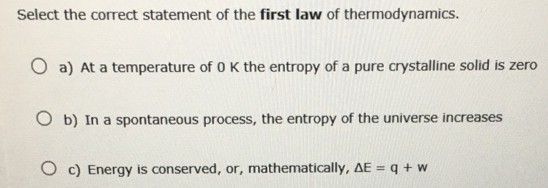# Problem: Select the correct statement of the first law of thermodynamics. a) At a temperature of 0 K the entropy of a pure crystalline solid isb) In a spontaneous process, the entropy of the universe increasesc) Energy is conserved, or, mathematically, delta E = q + w

🤓 Based on our data, we think this question is relevant for Professor Duim's class at UCD.

###### FREE Expert Solution

The First Law of Thermodynamics states that###### Problem Details

Select the correct statement of the first law of thermodynamics.

a) At a temperature of 0 K the entropy of a pure crystalline solid is

b) In a spontaneous process, the entropy of the universe increases

c) Energy is conserved, or, mathematically, delta E = q + w